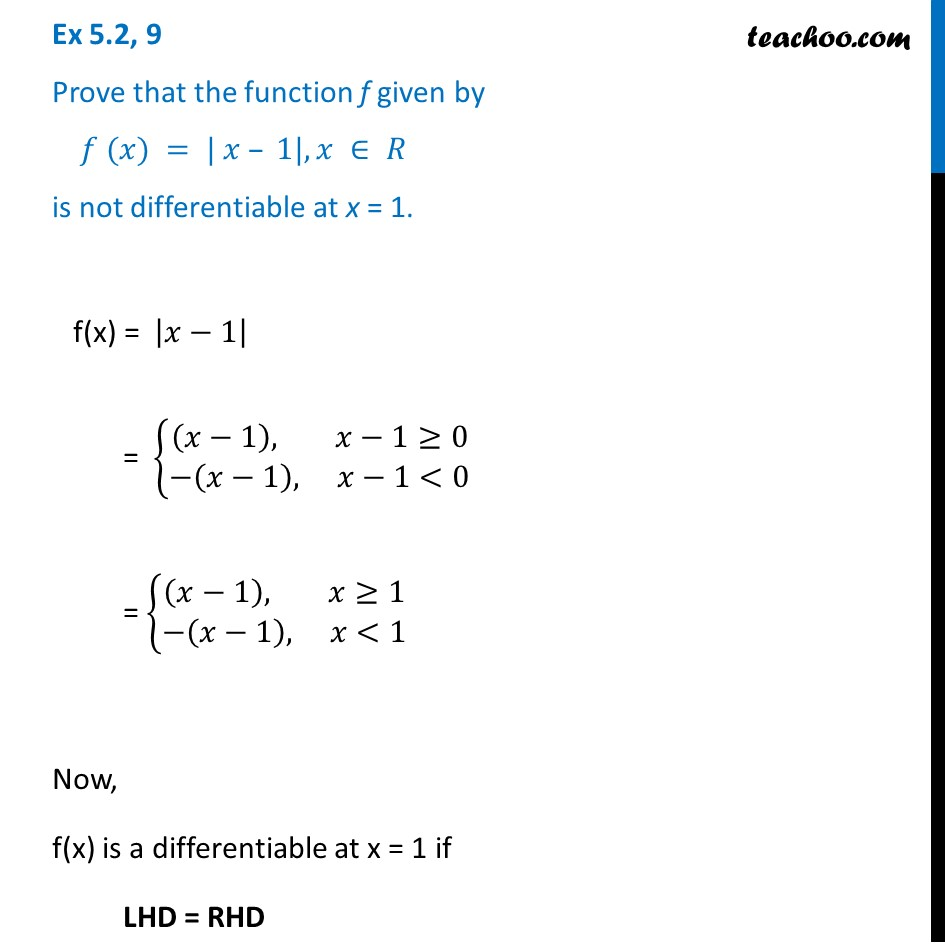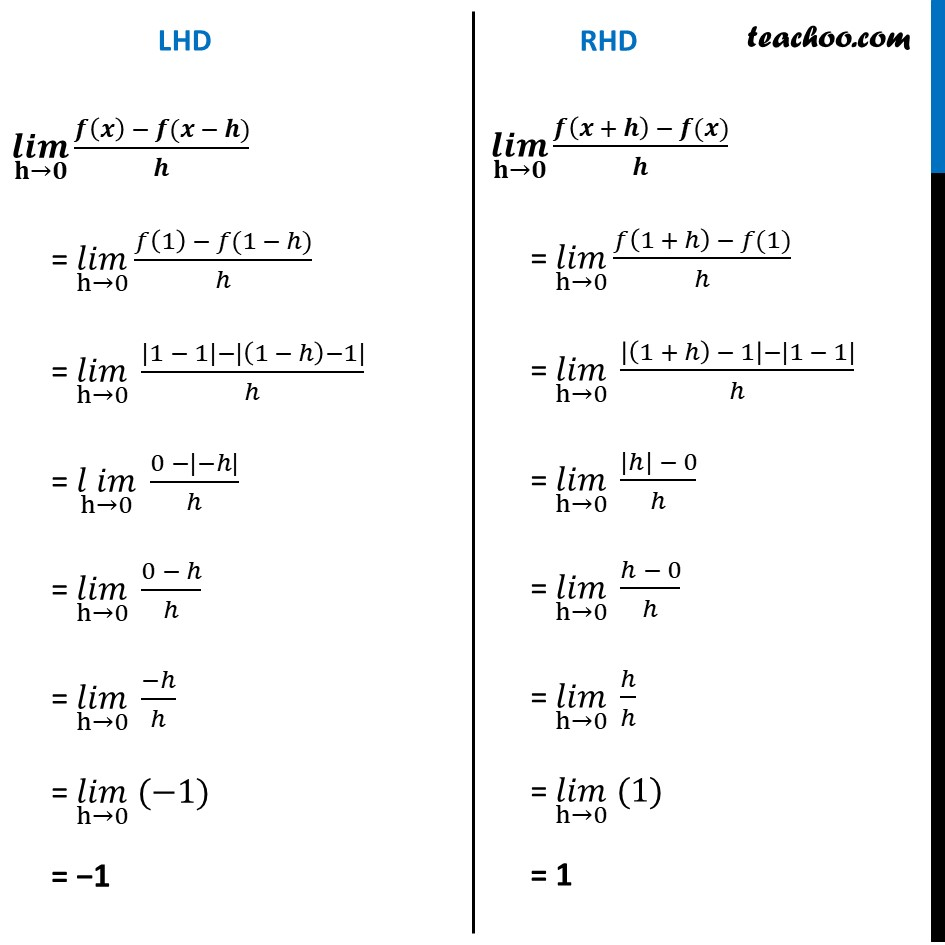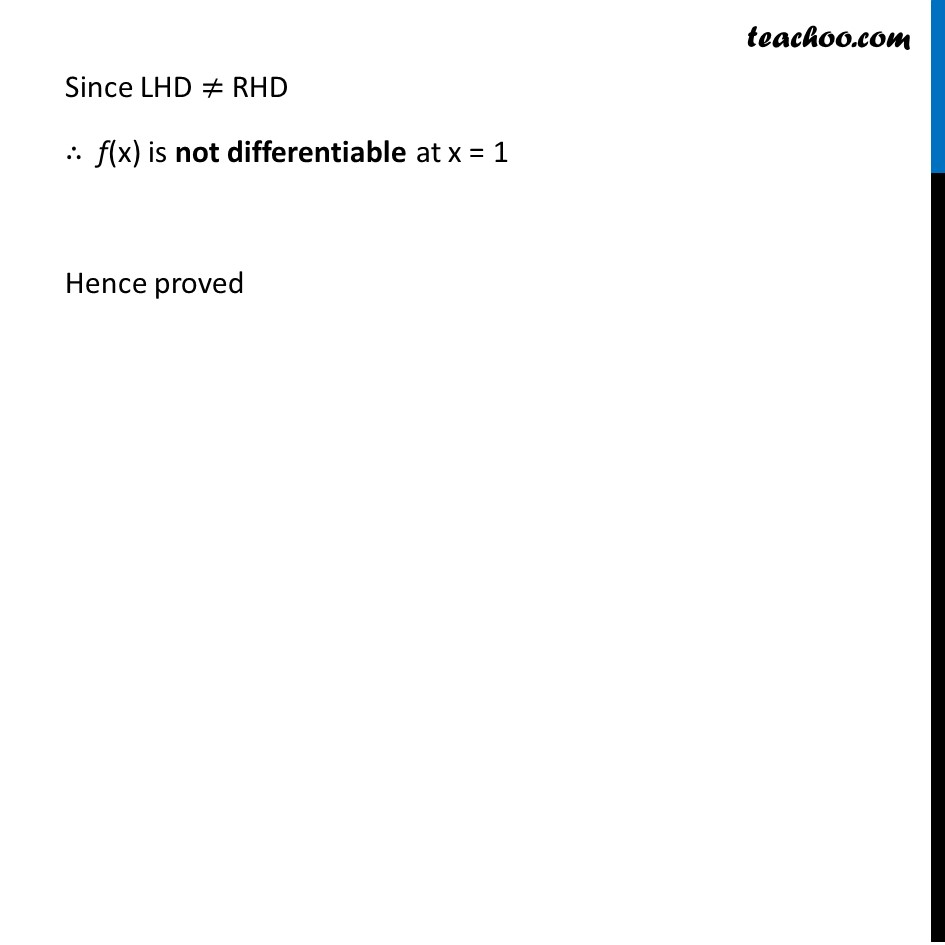Ex 5.2

Chapter 5 Class 12 Continuity and Differentiability
Serial order wiseLearn in your speed, with individual attention - Teachoo Maths 1-on-1 Class

### Transcript

Ex 5.2, 9 Prove that the function f given by 𝑓 (𝑥) = | 𝑥 – 1|, 𝑥 ∈ 𝑅 is not differentiable at x = 1. f(x) = |𝑥−1| = {█((𝑥−1), 𝑥−1≥0@−(𝑥−1), 𝑥−1<0)┤ = {█((𝑥−1), 𝑥≥1@−(𝑥−1), 𝑥<1)┤ Now, f(x) is a differentiable at x = 1 if LHD = RHD (𝒍𝒊𝒎)┬(𝐡→𝟎) (𝒇(𝒙) − 𝒇(𝒙 − 𝒉))/𝒉 = (𝑙𝑖𝑚)┬(h→0) (𝑓(1) − 𝑓(1 − ℎ))/ℎ = (𝑙𝑖𝑚)┬(h→0) (|1 − 1|−|(1 − ℎ)−1|)/ℎ = (𝑙 𝑖𝑚)┬(h→0) (0 −|−ℎ|)/ℎ = (𝑙𝑖𝑚)┬(h→0) (0 − ℎ)/ℎ = (𝑙𝑖𝑚)┬(h→0) (−ℎ)/ℎ = (𝑙𝑖𝑚)┬(h→0) (−1) = −1 (𝒍𝒊𝒎)┬(𝐡→𝟎) (𝒇(𝒙 + 𝒉) − 𝒇(𝒙))/𝒉 = (𝑙𝑖𝑚)┬(h→0) (𝑓(1 + ℎ) − 𝑓(1))/ℎ = (𝑙𝑖𝑚)┬(h→0) (|(1 + ℎ) − 1|−|1 − 1|)/ℎ = (𝑙𝑖𝑚)┬(h→0) (|ℎ| − 0)/ℎ = (𝑙𝑖𝑚)┬(h→0) (ℎ − 0)/ℎ = (𝑙𝑖𝑚)┬(h→0) ℎ/ℎ = (𝑙𝑖𝑚)┬(h→0) (1) = 1 Since LHD ≠ RHD ∴ f(x) is not differentiable at x = 1 Hence proved